# C263 – ASTM D3588/GPA 2145 – Calorific Value and Relative Density

## Description

This calculates the calorific value, compressibility factor and relative density of a natural gas mixture. The calculations are carried out at the standard US base temperature of 60°F.

ASTM D3588-98: Standard Practice for Calculating Heat Value, Compressibility Factor and Relative Density of Gaseous Fuels (1998)

GPA 2145: Table of Physical Properties for Hydrocarbons and Other Compounds of Interest to the Natural Gas Industry (1989, 2000, 2003, 2009)

GPA TP-17: Table of Physical Properties of Hydrocarbons for Extended Analysis of Natural Gases (1998)

Kelton calculation reference C263

FLOCALC calculation reference F067

KIMS calculation reference K202

Note: The BTU referred to in this calculation is the international table BTU, BTU = 1055.056 J.

## Options

### Gas Analysis

• Extended Analysis

This option allows common trace gases to be included in the analysis.

• Refinery trace gases

This option allows refinery trace gases to be included in the analysis.

### GPA 2145 Physical Constants Table

• 1989
• 2000
• 2003
• 2009

This option allows the user to select which revision of GPA 2145 they wish to take the table of physical constants, associated with the component gases, from.

### Wet Gas Correction

• Apply

This option allows the user to correct for wet gas

### Mole Percent of Water

• User entered
• Raoult’s law approximation

This allows the user to enter the mole percent of water or have it calculated according to Raoult’s Law.

## Constants

Gas constant – R = 8.314472 Jmol-1K-1

Vapour pressure of water – Pw_vap = 0.25636 psi

Base Temperature – Tb = 60°F

## Calculation

### Compressibility Factor

The compressibility factor is calculated by: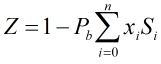Where Pb = Base pressure xi = Mole fraction of ith gas component Si = Summation factor of ith gas component

### Relative Density

The relative density is calculated by:

#### Ideal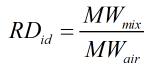#### Real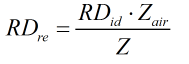Where MWmix = Molecular weight of gas mixture MWair = Molecular weight of air Z = Compressibility factor of gas mixture Zair = Compressibility factor of air

### Raoult’s Law approximation of water content

Assuming Raoult’s law, the water content when the gas is saturated will be: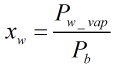### Volumetric Heating Value

The heating value is calculated using component ideal volumetric heating values if base pressure is equal to 14.696 psi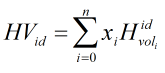If base pressure is not 14.696 psi the calculation multiplies the component ideal volumetric heating values by the pressure ratio (in psi) Pb/14.696 and then carries out the above calculation.

If there is a wet gas correction based on the Raoult’s law approximation then ideal volumetric heating value is calculated by: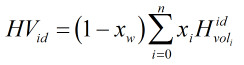Where xi = Mole fraction of ith gas component MWi = Molecular weight of ith gas component Hvolidi = Ideal volumetric gross heating value of ith gas component

These ideal heating values are divided by the compressibility factor to give a “real” heating value. This is not the real heating value but is actually the ideal heating value per real cubic foot.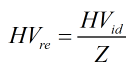Back